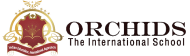Enquire Now

# Division Calculator

The result is:
What is Division?
The division is one of the basic mathematical arithmetic operations in which a larger number is broken into smaller groups and has the same number of items. It is the repetitive subtraction procedure and the inverse of the multiplication operation. It is the process of forming equal groups. It is represented by the two primary symbols "/" and "÷ ".

The Division calculator is a free and time-saving online tool. It is difficult to solve large numbers in less time. To make this problem hassle-free, you will find an easy and accurate way of getting division results in fractions of seconds. Does this calculator also support decimal numbers? The answer is Yes.

THE FORMULA FOR DIVISION
Dividend / Divisor = Quotient
The verification method of division can be expressed as
Dividend = (Divisor × Quotient) + Remainder
Here,
1. A dividend is the value that is being divided.
2. A divisor is a number by which the dividend is to be divided.
3. A quotient is the resultant of the division.
4. A remainder is the value that is left after division.
Example of Division
1. Find the quotient. 12 ÷ 3 =?
Here,
To perform addition in the basic calculator. Enter all the given values using the addition operator (+), i.e.,
82 + 65 = 147
2. Find the value.
67 + 85 + 99 + 76 + 119 + 34 = ?
To perform addition in the basic calculator. Enter all the given values using the addition operator (+), i.e.,
67 + 85 + 99 + 76 + 119 + 34 = 480
How to Use Division Calculator?
1. Enter the first value (Dividend).
2. Enter the second value (Divisor).
3. Click on the “Divide” button to get the result (Remainder and Quotient)

## FAQs

##### What is the divisibility rule of 11?

| K12 Techno Services ®

ORCHIDS - The International School | Terms | Privacy Policy | Cancellation